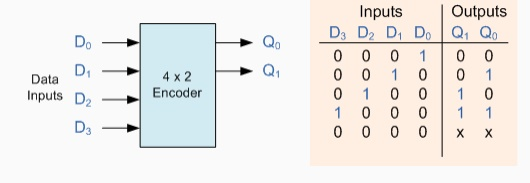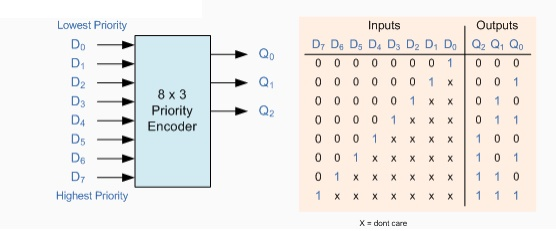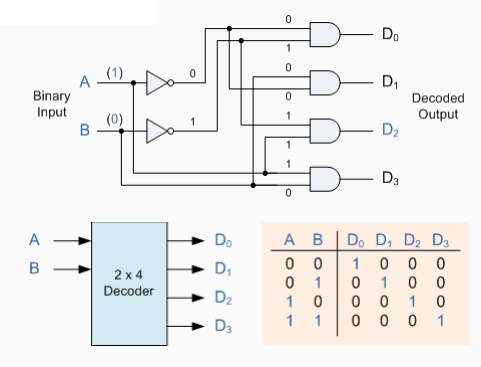# The Digital Encoder

Unlike a multiplexer that selects one individual data input line and then sends that data to a single output line or switch, a Digital Encoder more commonly called a Binary Encoder takes ALL its data inputs one at a time and then converts them into a single encoded output.

The Digital Encoder

Unlike a multiplexer that selects one individual data input line and then sends that data to a single output line or switch, a Digital Encoder more commonly called a Binary Encoder takes ALL its data inputs one at a time and then converts them into a single encoded output. So we can say that a binary encoder, is a multi-input combinational logic circuit that converts the logic level "1" data at its inputs into an equivalent binary code at its output. Generally, digital encoders produce outputs of 2-bit, 3-bit or 4-bit codes depending upon the number of data input lines. An "n-bit" binary encoder has 2n input lines and n-bit output lines with common types that include 4-to-2, 8-to-3 and 16-to-4 line configurations. The output lines of a digital encoder generate the binary equivalent of the input line whose value is equal to "1" and are available to encode either a decimal or hexadecimal input pattern to typically a binary or B.C.D. output code.

4-to-2 Bit Binary EncoderOne of the main disadvantages of standard digital encoders is that they can generate the wrong output code when there is more than one input present at logic level "1". For example, if we make inputs D1 and D2 HIGH at logic "1" at the same time, the resulting output is neither at "01" or at "10" but will be at "11" which is an output binary number that is different to the actual input present. Also, an output code of all logic "0"s can be generated when all of its inputs are at "0" OR when input D0 is equal to one.

One simple way to overcome this problem is to "Prioritise" the level of each input pin and if there was more than one input at logic level "1" the actual output code would only correspond to the input with the highest designated priority. Then this type of digital encoder is known commonly as a Priority Encoder or P-encoder for short.

Priority Encoders

Priority Encoders solve the problem mentioned above by allocating a priority level to each input. The encoder output corresponds to the currently active input with the highest priority. So when an input with a higher priority is present, all other inputs with a lower priority will be ignored. Priority encoders come in many forms with an example of an 8-input priority encoder along with its truth table shown below.

8-to-3 Bit Priority EncoderPriority encoders are available in standard IC form and the TTL 74LS148 is an 8 to 3 bit priority encoder which has eight active LOW (logic "0") inputs and provides a 3-bit code of the highest ranked input at its output. Priority encoders output the highest order input first for example, if input lines "D2", "D3" and "D5" are applied simultaneously the output code would be for input "D5" ("101") as this has the highest order out of the 3 inputs. Once input "D5" had been removed the next highest output code would be for input "D3" ("011"), and so on.

The Boolean expression for this 8-to-3 encoder above with inputs D0 to D7 and

outputs Q0, Q1, Q2 is given as:

Q0 = D1 + D3 + D5 + D7

Q1 = D2 + D3 + D6 + D7

Q2 = D4 + D5 + D6 + D7

Then the implementation of these Boolean expression outputs above using individual OR gates is as follows.

Digital Encoder using Logic GatesBinary Decoder

A Decoder is the exact opposite to that of an "Encoder" we looked at in the last tutorial. It is basically, a combinational type logic circuit that converts the binary code data at its input into one of a number of different output lines, one at a time producing an equivalent decimal code at its output. Binary Decoders have inputs of 2-bit, 3-bit or 4-bit codes depending upon the number of data input lines, and a n-bit decoder has 2n output lines. Therefore, if it receives n inputs (usually grouped as a binary or Boolean number) it activates one and only one of its 2n outputs based on that input with all other outputs deactivated. A decoders output code normally has more bits than its input code and practical binary decoder circuits include, 2-to-4, 3-to-8 and 4-to-16 line configurations.

A binary decoder converts coded inputs into coded outputs, where the input and output codes are different and decoders are available to "decode" either a Binary or BCD (8421 code) input pattern to typically a Decimal output code. Commonly available BCD-to-Decimal decoders include the TTL 7442 or the CMOS 4028. An example of a 2-to-4 line decoder along with its truth table is given below. It consists of an array of four NAND gates, one of which is selected for each combination of the input signals A and B.

A 2-to-4 Binary Decoders.In this simple example of a 2-to-4 line binary decoder, the binary inputs A and B determine which output line from D0 to D3 is "HIGH" at logic level "1" while the remaining outputs are held "LOW" at logic "0" so only one output can be active (HIGH) at any one time. Therefore, whichever output line is "HIGH" identifies the binary code present at the input, in other words it "de-codes" the binary input and these types of binary decoders are commonly used as Address Decoders in microprocessor memory applications.Some binary decoders have an additional input labelled "Enable" that controls the outputs from the device. This allows the decoders outputs to be turned "ON" or "OFF" and we can see that the logic diagram of the basic decoder is identical to that of the basic demultiplexer. Therefore, we say that a demultiplexer is a decoder with an additional data line that is used to enable the decoder. An alternative way of looking at the decoder circuit is to regard inputs A, B and C as address signals. Each combination of A, B or C defines a unique address which can access a location having that address.

Sometimes it is required to have a Binary Decoder with a number of outputs greater than is available, or if we only have small devices available, we can combine multiple decoders together to form larger decoder networks as shown. Here a much larger 4-to-16 line binary decoder has been implemented using two smaller 3-to-8 decoders.

A 4-to-16 Binary Decoder Configuration.Inputs A, B, C are used to select which output on either decoder will be at logic "1" (HIGH) and input D is used with the enable input to select which encoder either the first or second will output the "1

BCD to 7-Segment Display Decoder

As we saw in the previous tutorial, a Decoder IC, is a device which converts one digital format into another and the most commonly used device for doing this is the Binary Coded Decimal (BCD) to 7-Segment Display Decoder. 7-segment LED (Light Emitting Diode) or LCD (Liquid Crystal) displays, provide a very convenient way of displaying information or digital data in the form of numbers, letters or even alpha-numerical characters and they consist of 7 individual LED's (the segments), within one single display package.

In order to produce the required numbers or HEX characters from 0 to 9 and A to F respectively, on the display the correct combination of LED segments need to be illuminated and BCD to 7-segment Display Decoders such as the 74LS47 do just that. A standard 7-segment LED display generally has 8 input connections, one for each LED segment and one that acts as a common terminal or connection for all the internal segments. Some single displays have an additional input pin for the decimal point in their lower right or left hand corner.

There are two important types of 7-segment LED digital display.

The Common Cathode Display (CCD) - In the common cathode display, all the cathode connections of the LED's are joined together to logic "0" and the individual segments are illuminated by application of a "HIGH", logic "1" signal to the individual Anode terminals.

The Common Anode Display (CAD) - In the common anode display, all the anode connections of the LED's are joined together to logic "1" and the individual segments are illuminated by connecting the individual Cathode terminals to a "LOW", logic "0" signal.

7-Segment Display FormatTruth Table for a 7-segment display7-Segment Display Elements for all Numbers.

It can be seen that to display any single digit number from 0 to 9 or letter from A to F, we would need 7 separate segment connections plus one additional connection for the LED's "common" connection. Also as the segments are basically a standard light emitting diode, the driving circuit would need to produce up to 20mA of current to illuminate each individual segment and to display the number 8, all 7 segments would need to be lit resulting a total current of nearly 140mA, (8 x 20mA). Obviously, the use of so many connections and power consumption is impractical for some electronic or microprocessor based circuits and so in order to reduce the number of signal lines required to drive just one single display, display decoders such as the BCD to 7-Segment Display Decoder and Driver IC's are used instead.

Binary Coded Decimal

Binary Coded Decimal (BCD or "8421" BCD) numbers are made up using just 4 data bits (a nibble or half a byte) similar to theHexadecimal numbers we saw in the binary tutorial, but unlike hexadecimal numbers that range in full from 0 through to F, BCD numbers only range from 0 to 9, with the binary number patterns of 1010 through to 1111 (A to F) being invalid inputs for this type of display and so are not used as shown below.BCD to 7-Segment Display Decoders

A binary coded decimal (BCD) to 7-segment display decoder such as the TTL 74LS47 or 74LS48, have 4 BCD inputs and 7 output lines, one for each LED segment. This allows a smaller 4-bit binary number (half a byte) to be used to display all the denary numbers from 0 to 9 and by adding two displays together, a full range of numbers from 00 to 99 can be displayed with just a single byte of 8 data bits.

BCD to 7-Segment DecoderThe use of packed BCD allows two BCD digits to be stored within a single byte (8-bits) of data, allowing a single data byte to hold a BCD number in the range of 00 to 99.

Study Material, Lecturing Notes, Assignment, Reference, Wiki description explanation, brief detail
Basic Electrical and Electronics Engineering : Digital Electronics : The Digital Encoder |